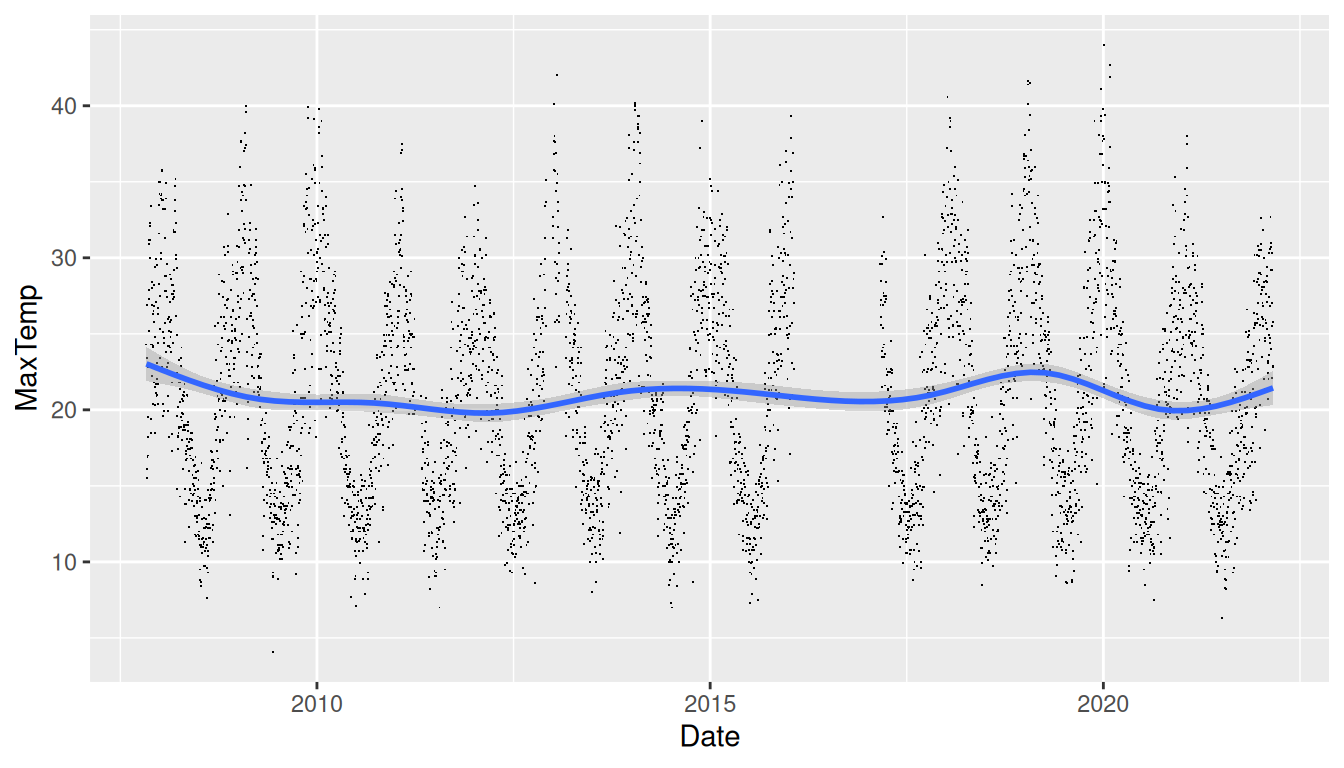## 11.50 Scatter Plot Smooth Gam

REVIEWds %>%
filter(location=="Canberra") %>%
ggplot(aes(x=date, y=max_temp)) +
geom_point(shape=".") +
geom_smooth(method="gam", formula=y~s(x, bs="cs")) +
labs(x=vnames["date"], y=vnames["max_temp"])

This scatter plot of x=date against y=max_temp shows a pattern of seasonality over the dataset and a trend line over the period of the dataset.

The scatter plot is again created using ggplot2::geom_point(). Typical of scatter plots of big data there will be many overlaid points. To reduce the impact the points are reduced to a small dot using shape=".".

An additional layer of a smooth fitted curve, using ggplot2::geom_smooth(), is added. The dataset has many points and so a smoothing method recommended is method="gam" which will automatically be chosen if not specified but with a message to that effect. The formula specified using formula= is also the default for method="gam".

Your donation will support ongoing development and give you access to the PDF version of this book. Desktop Survival Guides include Data Science, GNU/Linux, and MLHub. Books available on Amazon include Data Mining with Rattle and Essentials of Data Science. Popular open source software includes rattle, wajig, and mlhub. Hosted by Togaware, a pioneer of free and open source software since 1984.